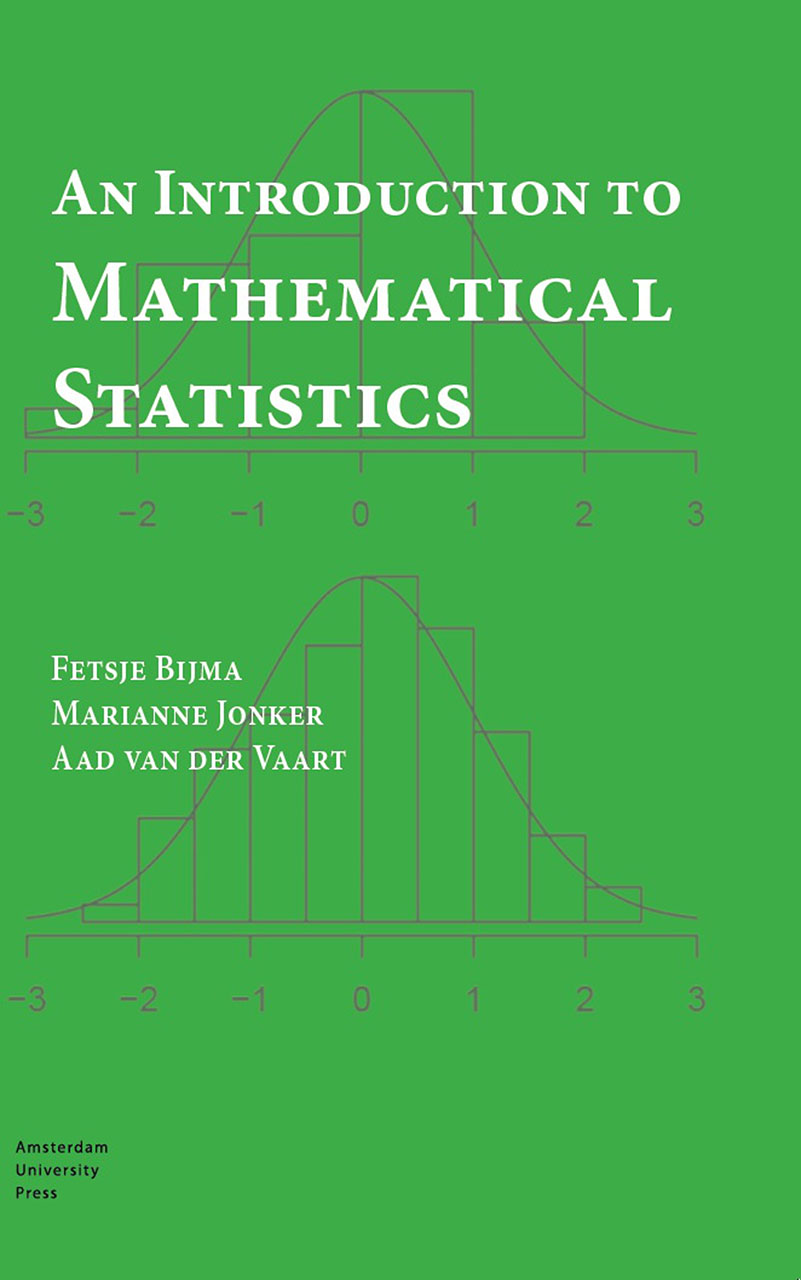Title
An Introduction to Mathematical Statistics
Translator
Reinie Erné
Price
€ 34,99
ISBN
9789462985100
Binding
Paperback
Number of pages
384
Publication date
Dimensions
24 x 17 cm
Also available as
eBook PDF - € 34,99

# An Introduction to Mathematical Statistics

Statistics is the science that focuses on drawing conclusions from data, by modeling and analyzing the data using probabilistic models. In An Introduction to Mathematical Statistics the authors describe key concepts from statistics and give a mathematical basis for important statistical methods. Much attention is paid to the sound application of those methods to data.

The three main topics in statistics are estimators, tests, and confidence regions. The authors illustrate these in many examples, with a separate chapter on regression models, including linear regression and analysis of variance. They also discuss the optimality of estimators and tests, as well as the selection of the best-fitting model.

Each chapter ends with a case study in which the described statistical methods are applied. This book assumes a basic knowledge of probability theory, calculus, and linear algebra.

€ 34,99
+ Order this book
Authors

# Fetsje Bijma

Fetsje Bijma worked as an assistant professor of mathematics at the Vrije Universiteit Amsterdam for 10 years. She studied mathematics at the University of Groningen and received a Ph.D. at the Faculty of Medicine of the Vrije Universiteit Amsterdam. She now works in industry.

# Marianne Jonker

Marianne Jonker is a biostatistician at the Radboud University Medical Center in Nijmegen. She studied mathematics and received her Ph.D. at the Vrije Universiteit Amsterdam, where she worked as an assistant professor in mathematics for some time. She has done work as a biostatistician for the Leiden University Medical Center in Leiden and the VU University Medical Center in Amsterdam.